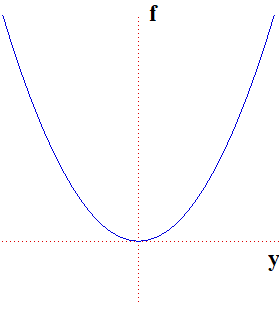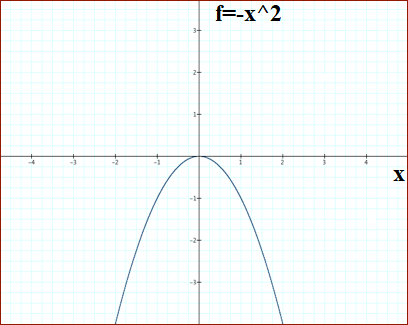# Find the (one) critical point (a,b) of the function f(x,y)=y^2-x^2. Sketch the cross sections...

## Question:

Find the (one) critical point {eq}(a,b) {/eq} of the function {eq}f(x,y)=y^2-x^2 {/eq}. Sketch the cross sections obtained by setting {eq}x=a {/eq} and {eq}y=b {/eq}. Do you think {eq}(a,b) {/eq} corresponds to a local extremum? Why or why not? (include a sketch of the cross section)

(Hint: If you adjust the {eq}x {/eq} coordinate of the critical point, does the output of {eq}f {/eq} go up or down? How about when you adjust the {eq}y {/eq} coordinate?)

## Critical Points:

For the function of two or more variables, the critical points are found in the manner similar to that for the function of one variable. The function has a local extremum if the value of the function will decrease/increase when we step away from the critical point in any direction. If it increases, we have a local minimum, if it decreases, we have a local maximum. The necessary condition for the function to have an extermum is to have zero partial derivatives at a given point.

The critical point of a function is determined by solving the following system of equations:

{eq}\begin{cases} \dfrac {\partial f}{\partial x} = 0 \\ \dfrac {\partial f}{\partial y} = 0 \end{cases} {/eq}

Taking the appropriate derivatives, we obtain:

{eq}\begin{cases} -2x = 0 \\ 2y = 0 \end{cases} {/eq}

The only critical point is the point {eq}(0, 0) {/eq}. The cross-sections of the function look like this:

a) {eq}x = 0 {/eq}

{eq}f (0, y) = y^2 {/eq}b) {eq}y = 0 {/eq}

{eq}f(x, 0) = - x^2 {/eq}This critical point does NOT correspond to a local extremum. The function will increase if we adjust y-coordinate from the critical point, keeping x zero, but will decrease if we do the same with x-coordinate. Therefore, we have a saddle point at the critical point.